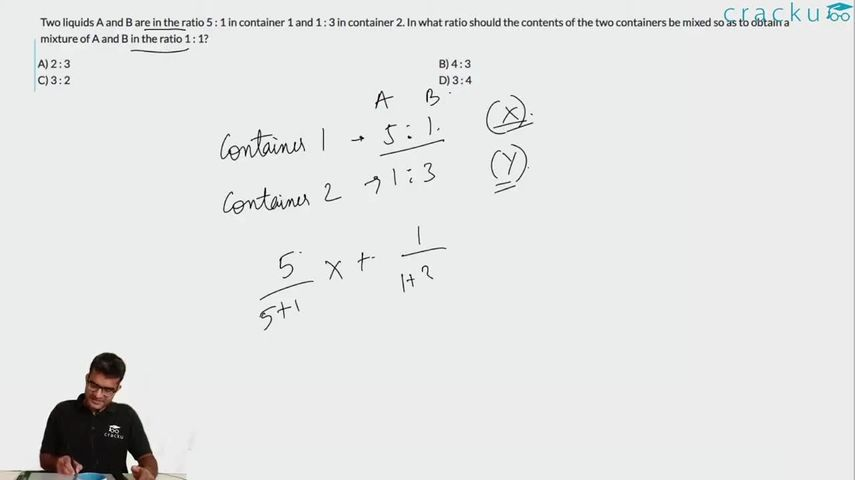Question 32

# Two liquids A and B are in the ratio 5 : 1 in container 1 and 1 : 3 in container 2. In what ratio should the contents of the two containers be mixed so as to obtain a mixture of A and B in the ratio 1 : 1?

Solution

Fraction of A in contained 1 = $$\frac{5}{6}$$

Fraction of A in contained 2 = $$\frac{1}{4}$$

Let the ratio of liquid required from containers 1 and 2 be x:1-x

x($$\frac{5}{6}$$) + (1-x)($$\frac{1}{4}$$) = $$\frac{1}{2}$$

$$\frac{7x}{12}$$ = $$\frac{1}{4}$$

=> x = $$\frac{3}{7}$$

=> Ratio = 3:4

### View Video Solution